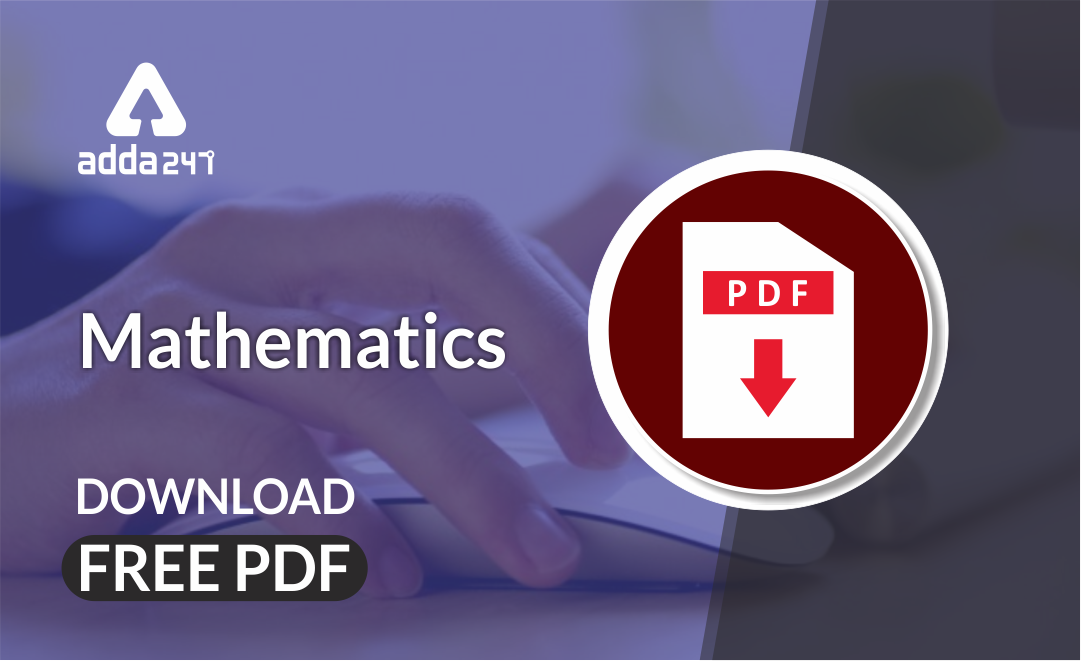# Algebra based Mathematics Notes For CTET Exam : Free PDFMathematics is an equally important section for CTET, MPTET, KVS & DSSSB Exams and has even more abundant importance in some other exams conducted by central or state govt. Generally, there are questions asked related to basic concepts, Facts and Formulae of the Algebra.

To let you make the most of Mathematics section, we are providing important facts related to the Algebra. At least 2-3 questions are asked from this topic in most of the teaching exams. We wish you all the best of luck to come over the fear of the Mathematics section.

How to Overcome Exam Fever, Especially When You Fear Maths

## Algebra

Algebra

It is branch of mathematics that substitutes letters for numbers. Algebra gives different methods of solve equations.

Variables

A variable is represented by either a sign or a letter. Its value may not be the same in every equation.

Example: 2x – 10 = 0; here, will get x = 5

3x – 3 = 0; here, he will get x = 10.

Here, x can have different values in different equations.

Therefore, x is variable

Constants

A constant always has fixed values. Every real number is a constant.

Example: 2, 5, 7 etc.

Expressions

An expression is a combination of constant and/or variables connected to each other by mathematical operators (addition, subtraction, multiplication and division).

Example: 3x + 5, 2y² – 4x 5, etc.

Terms

The parts of an expression are separated from one another by plus or minus sign and are called terms of that expression.

Example: in 3x  + 5, 3x and 5 are both expressions, as they are separated by a plus sign.

In 2y² – 4x + 5, 2y², 4x and 5 are separated by plus and minus signs, Therefore, they all are terms.

Like Terms

Two or more terms are said to be alike if their algebraic factors are the same.

Example: 3x²y, 7x²y and 10x²y are like terms.

In the expression 2xy + 3x – 4y  – 7xy, 2xy and 7xy are like terms.

Unlike Terms

Two or more terms are said to be unalike if their algebraic factors are different

Example: 3x²y, 7xy and 10xy² are unlike terms.

Factors

When numbers and variables multiply to form a product, each quantity is called a factor of the product.

Example: Factors of 3xy are 3, x and y.

Coefficients

The numerical part of term is called its coefficient.

Example: In 6x³, 6 is the coefficient of x³.

Polynomials

The algebraic expression having one or more terms, each of which consists of a constant multiplied by one or more variables raised to a negative integral power, is called a polynomial.

A polynomial can have any finite number of terms.

Monomials, binomials and trinomials are the types of polynomials.

Monomials

The algebraic expression having a single term is called a monomial.

Example: 4x is a monomial expression.

Binomials

The algebraic expression having two terms is called a binomial.

Example: 4x³ + 7x is binomial expression

Trinomials

The algebraic expression having three terms is called a trinomial.

Example: 4x³ + 2y² – x is a trinomial expression.

Addition and subtraction of Algebraic Expressions

The addition or subtraction of algebraic expressions can be simplified by combining the like terms. In this method, coefficient or combined according to their signs, keeping the same algebraic factors.

Example:= 9x²y + xy²

Multiplication of Algebraic Expressions

By the distributive law, the product of algebraic expressions is calculated.

Example:

1. Find 2x²y × (7x²y – 2xy²).

We have

2x²y × (7x²y – 2xy²)

= 2x²y × 7x²y – 2x²y × 2xy²

= 14x⁴y² – 4x³y³